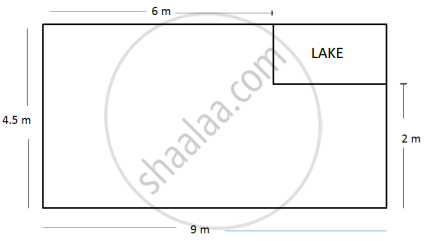# A missing helicopter is reported to have crashed somewhere in the rectangular region shown in the figure. What is the probability that it crashed inside the lake shown in the figure? - Algebra

Sum

A missing helicopter is reported to have crashed somewhere in the rectangular region shown in the figure. What is the probability that it crashed inside the lake shown in the figure?#### Solution

The helicopter is equally likely to crash anywhere in the region. In the figure, the length and the breadth of the rectangle are 9 m and 4.5 m respectively.

Area of the entire region where the helicopter can crash

= (9 × 4.5) m2 = 40.5 m2

Let A be the event that helicopter crashed inside the lake.

The lake is rectangular shaped.

Length of lake = 9 – 6 = 3 m

Breadth of lake = 4.5 – 2 = 2.5 m

Area of lake = length × breadth

= 3 × 2.5

= 7.5 m2

∴ P(A) = ("n"("A"))/("n"("S"))

= 7.5/40.5

= 75/405

= 5/27

The probability that the helicopter crashed inside the lake is 5/27

Concept: Probability of an Event
Is there an error in this question or solution?